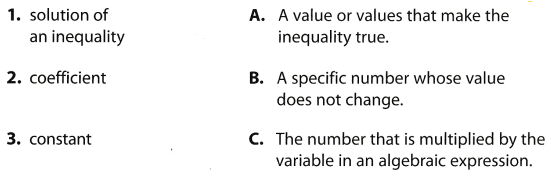# Texas Go Math Grade 6 Module 13 Answer Key Inequalities and Relationships

Refer to our Texas Go Math Grade 6 Answer Key Pdf to score good marks in the exams. Test yourself by practicing the problems from Texas Go Math Grade 6 Module 13 Answer Key Inequalities and Relationships.

## Texas Go Math Grade 6 Module 13 Answer Key Inequalities and Relationships

Write an integer to represent each situation.

Question 1.
a loss of $75 Answer: Because here we have loss, we will have -, so, the required integer is 75. Question 2. a football player’s gain of 9 yards Answer: Here, because we have gain, we will have +. so, the required integer is 9. Question 3. spending$1,200 on a flat screen TV __________
Because here we have spending, we will have -, so the required integer is -1.200.

Question 4.
a climb of 2,400 feet _________
Because here we have climbing, we will have +, so the required integer is 2.400.

Find the product or quotient.

Question 5.
6 × 9 __________
Here, the signs of the integers are the same, so, the product will be positive. We have that result is 54.

Question 6.
15 ÷ (-5) ________
Here, the signs of integers are different, so the quotient will be negative.
We have that result is -3.

Question 7.
-8 × 6 _______
Here, the signs of integers are different, so the product will be negative.
So, the result is -48.

Question 8.
-100 ÷ (10) _________
Here, the signs of integers are different, so the quotient will be negative.
We have that result is -10.

Question 9.
3 × (-7) ________
Here, the signs of integers are different, so the product will be negative.
So, the result is -21.

Question 10.
-64 ÷ 8 ________
Here, the signs of integers are different, so the quotient will be negative.
We have that result is -8.

Question 11.
-8 × (-2) __________
Here, the signs of the integers are the same, so, the product will be positive We have that result is 16.

Question 12.
32 ÷ 2 ___________
Here, the signs of the integers are the same, so, the quotient will be positive. We have that result is 16.

Solve.

Question 13.
9p = 108 __________
Here we have that p is multiplied by 9, so, we will multiply both sides by the reciprocal, $$\frac{1}{9}$$, in order to isolate variable. After that we will simplify and get the following:
$$\frac{1}{9}$$ ∙ 9p = 108 ∙ $$\frac{1}{9}$$
p = $$\frac{108 \cdot 1}{9}$$
p = 12

Question 14.
$$\frac{3}{5}$$n = 21 _________
3 5
Here we have that n is multiplied by $$\frac{3}{5}$$, so, we will multiply both sides by the reciprocal, $$\frac{3}{5}$$, in order to isolate the variable.
After that we will simplify and get the following:
$$\frac{5}{3}$$ ∙ $$\frac{3}{5}$$n = 21 ∙ $$\frac{5}{3}$$
n = $$\frac{21 \cdot 5}{3}$$
n = 35

Question 15.
$$\frac{4}{7}$$k = 84 __________
$$\frac{7}{4}$$ ∙ $$\frac{4}{7}$$k = 84 ∙ $$\frac{7}{4}$$
k = $$\frac{84 \cdot 7}{4}$$
k = 147

Question 16.
$$\frac{3}{20}$$ e = 24 ___________
$$\frac{20}{3}$$ ∙ $$\frac{3}{20}$$e = 24 ∙ $$\frac{20}{3}$$
e = $$\frac{24 \cdot 20}{3}$$
e = 160

Visualize Vocabulary

Use the ✓ words to complete the graphic.Understand Vocabulary

Match the term on the left to the correct expression on the right.